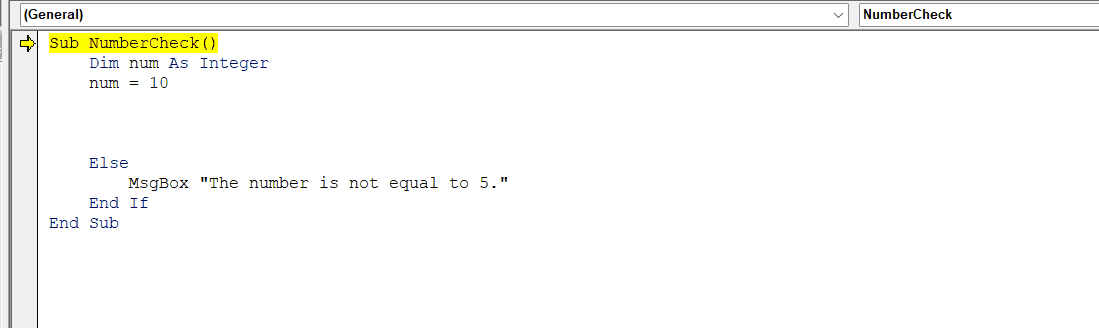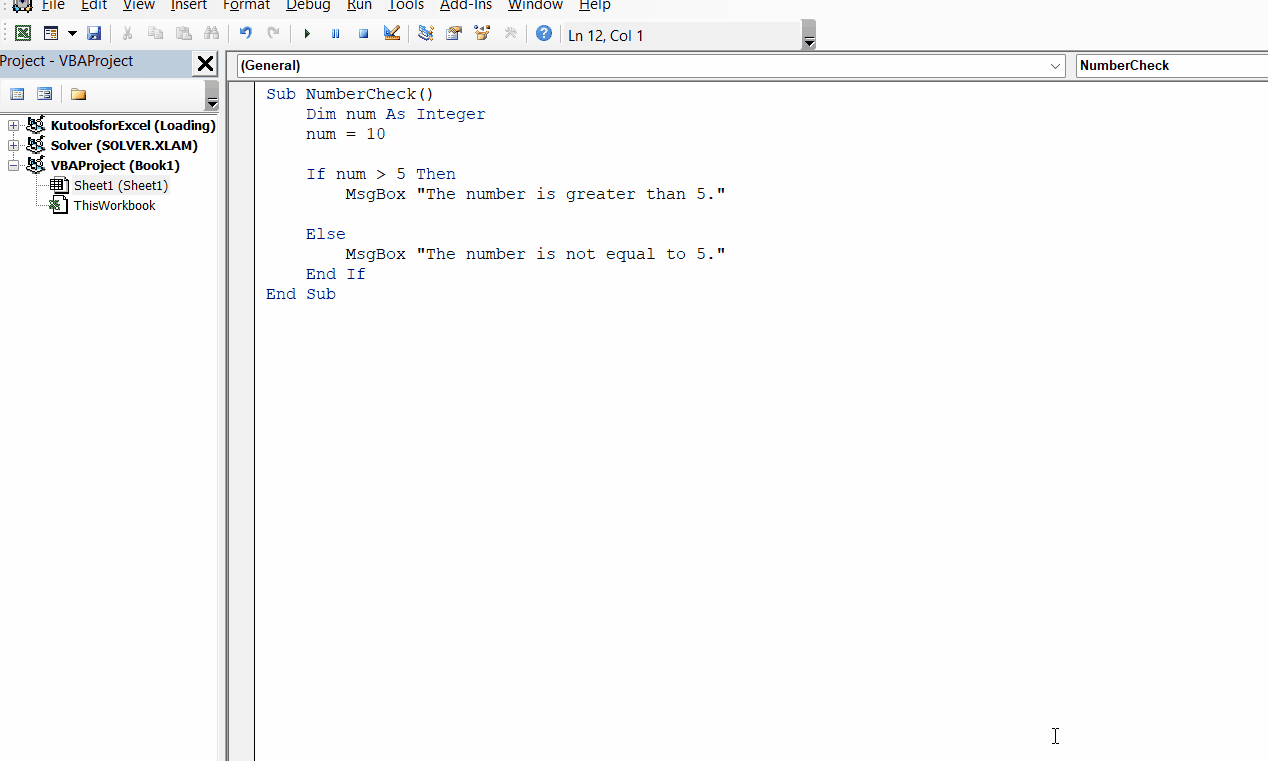# “ Else without If ” error in Microsoft Excel

In this article, we will learn how to cater to the “Else without If error” in Microsoft Excel. To avoid this error, we simply need to provide a preceding If statement to the Else statement. Such that, the If statement contains the original action and the Else statement contains the alternative action.

The correct syntax for an If-Else statement is:

For instance, we have a basic VBA code to check if a number is more significant or lesser than 5. We are facing the “ Else without If ” error.

In the following steps, we will address or fix the error.

The “Else without If” error in Microsoft Excel arises when VBA code contains an Else statement without a matching If statement. This error message indicates a syntax issue that is to be resolved utilizing the correct syntax.

### Step 1 – Input the If Statement– Input the If statement prior to the Else statement to complete the syntax.
– The If statement will be:

If num > 5 Then
MsgBox “The number is greater than 5.”

– The “num>5” is the condition and  “The number is greater than 5.”  is the statement to be returned if the condition is true.
– The complete code in this case would be:

Sub NumberCheck()
Dim num As Integer
num = 10

If num > 5 Then
MsgBox “The number is greater than 5.”

Else
MsgBox “The number is not equal to 5.”
End If
End Sub

### Step 2 – Run the Code– We will run the code to check wether the problem is fixed.